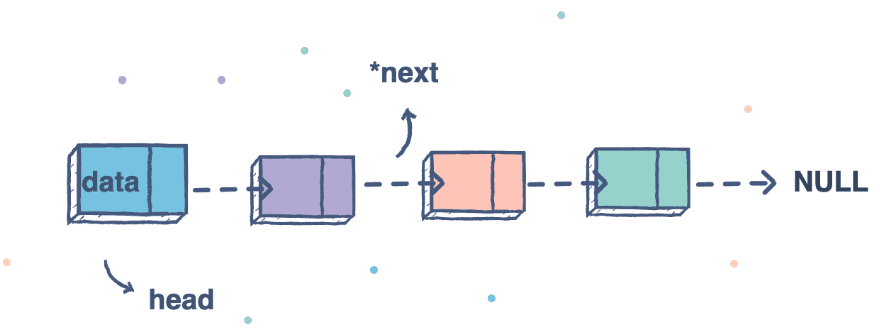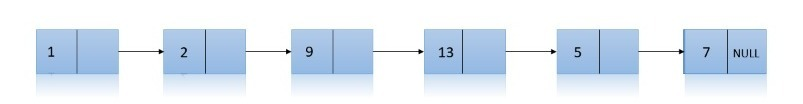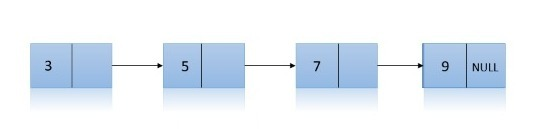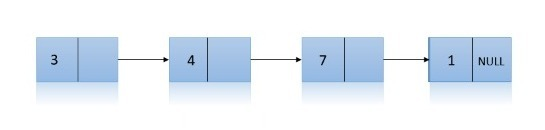## Introduction

In this blog, we will discuss a problem that comes in the favorite list of Interviewers.

The problem is to check Identical Linked Lists.

First, let us see what a linked list is and how we can create a linked list.

linked list is a linear data structure consisting of nodes where each node points to the next node using a pointer. Each node is composed of data and a pointer to the next node.Structure of a Node in a linked list:

``````struct Node
{
int key;
Node(int x){
key=x;
next=NULL;
}
struct Node *next;
};``````

To sort a linked list of 0s,1s, and 2s, we need to return the head of the linked list.

## Some Examples

Input 1:Output 1:

Both lists are Identical

Input 2:Output 2:

Both lists are Not Identical

## Iterative Approach

1. Create a boolean function checkIdenticalLists() which takes two parameters one is the head of the first linked list, and the other is the head of another linked list and store the heads of both the linked lists in two pointers, for example, Node *curr1 = head1, *curr2 = head2;
2. Now, traverse both the linked list simultaneously and compare the data of nodes of both the linked list at every point, and if at any moment the data of a specific node of the linked lists are not the same, then return false from the function else continue.
3. After completing the loop, we have to check if the length of the linked lists is the same or not and to do so. We have to check if the value of both the pointers is the same.
4. After that return true from the function checkIdenticalLists().

### Code in C++

``````// C++ Program to check Identical Linked Lists
#include <bits/stdc++.h>
using namespace std;

struct Node
{
int val;
Node(int x)
{
val = x;
next = NULL;
}
struct Node *next;
};
// Function to check Identical Linked lists
{

// traversing both the linked list simultaneously
while (curr1 != NULL && curr2 != NULL)
{
if (curr1->val != curr2->val) // if at any point the value of nodes of both the linked list is not same then return false
{
return false;
}
curr1 = curr1->next;
curr2 = curr2->next;
}

if (curr1 != curr2) // checking if both the linked list have same length if not then also returning false
{
return false;
}
// else the linked lists are identical return true
return true;
}

// Function to insert a node
Node *insert(Node *x, int y)
{
Node *temp = new Node(y);
temp->next = x;
return temp;
}

// Driver code
int main()
{
{
cout << "Both linked lists are identical" << endl;
}

else
{
cout << "Both linked lists are not identical" <<endl;
}
return 0;
}``````

``````Input:
List1: 10->5->4->11->9
List2: 10->5->4->11->9

Output:

### Complexity Analysis

Time Complexity: O(n) (Worst Case)

Since there are n nodes in the linked list and we are doing traversals of the whole linked list, therefore, the time complexity is O(n)

Space Complexity: O(1) (Worst case)

Since we are not using any data structures other than the linked list given to us, therefore, the space complexity is O(1)

## Recursive Approach

1. Create a boolean function checkIdenticalLists(), which takes two parameters; one is the head of the first linked list, and the other is the head of another linked list.
2. Add base case condition to check if the current node of the linked list is NULL or not.
3. Compare the current values of the nodes of the linked list and simultaneously make a recursive call for the function; after the call is complete, .it will return true if both the conditions are true, else it will return false.
4. To tackle the case where the different lengths of the linked lists are given, add one extra statement of return false outside the if condition stated in the previous step.

### Code in C++

``````// C++ Program to check Identical Linked Lists
#include <bits/stdc++.h>
using namespace std;

struct Node
{
int val;
Node(int x)
{
val = x;
next = NULL;
}
struct Node *next;
};

// Function to check Identical Linked lists
{
// we reach the end of both the linked lists, therefore, return true
{
return true;
}

//checking for the primary condition
{
// check the value of the current node and also check it recursively for every other node in the linked lists

}
// One of the lists is empty; therefore, return false
return false;
}

// Function to insert a node
Node *insert(Node *x, int y)
{
Node *temp = new Node(y);
temp->next = x;
return temp;
}

// Driver code
int main()
{
{
cout << "Both linked list are identical" << endl;
}
else
{
cout << "Both linked lists are not identical" <<endl;
}
return 0;
}``````

``````Input:
List1: 1->5->0->7->3
List2: 1->5->0->3

Output:
Both linked lists are not identical.``````

### Complexity Analysis

Time Complexity: O(n) (Worst Case)

Since there are n nodes in the linked list and we are doing traversals of the whole linked list, therefore, the time complexity is O(n)

Space Complexity: O(N) (Worst case)

Since we are making recursive calls there some stack is used it approximately equal to O(n)

Q1. What is a doubly-linked list?

It is a linked list containing pointers to both the previous and next node and has the node's value.

1. Memory usage in a linked list is more than an array.
2. Reverse traversals are not possible in the linked list.
3. Random access is not possible in a linked list.

Q3. What are the applications of linked lists?

1. They are used in the Implementation of stacks and queues.
2. Linked lists are also used to implement graphs, i.e., Adjacency list representation of the graph.
3. Arithmetic operations on large integers are done using a linked list.

## Key takeaways

In this article, we discussed how we could check if two linked lists are identical or not. We discussed two linked approaches; one was using iterative traversal and the other was using recursive traversal.

We hope you gained some insight into this problem, and now it is your turn to practice this problem and code it out in your way.

Don't Stop here. Try more problems in the linked list and gain expertise!

Until then, Keep Learning and Keep Coding.,